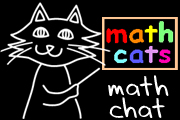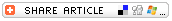## Search form# What Is Math?

## By Wendy PettiWhat is math? It might seem obvious: We hope we know what we're teaching -- and that our students know what they're learning! But responses to that question can be surprisingly diverse.

Before you read on, please take a few minutes to reflect and record your own thoughts on "What is math?"Illustration courtesy of Wendy Petti.

I'm a math educator -- I teach grade 4 math, I've created a math Web site, and I write about topics in math education. Yet I cannot easily express what math is. I'm in good company. Bertrand Russell has quipped, "Mathematics may be defined as the subject in which we never know what we are talking about, nor whether what we are saying is true." Math teacher Sanderson M. Smith observes that "students can gain a tremendous appreciation for mathematics if they understand that the question 'What is mathematics?' has been analyzed and debated since the time of the Pythagoreans, around 550 B.C. "

It is worth pursuing a clear understanding of the meaning and scope of mathematics so that we might provide our students with a richer learning experience and help them more fully appreciate the beauty and power of mathematics.

## STUDENTS AND TEACHERS REFLECT ON "WHAT IS MATH?"

In my math classes, no matter how much attention we pay to the five content standards and five process standards, many of my students seem to focus on number and operation when they share their thoughts and feelings about math. One of my students had an "aha!" moment the morning after a student-led math night: "I look at math a different way now. I've usually thought of math as something we need to do, to know how much money we need to pay, things like that. But this morning I woke up and said, 'Wow! Math is everywhere!'"

A 2005 Math Cats writing contest on "What is math?" produced these reflections:

• From an elementary school volunteer: "Math is more than a subject we learn in school. Math is every breath we take and every second of the day. From the moment we wake up in the morning, math is the core of everything we do..."
"Math is you.
Math is me.
Math is everything we see!
Infinity and beyond our wildest dreams
Math encompasses all extremes!"
• From a 13-year-old: "Math is the entire world simplified on a piece of paper... Math is ingeniousness morphed into a tiny simple formula so we can harness its fantastic powers."
• From a 12-year-old: "Math is the universal language of the world."
• From an 11-year-old: "No one can live without math; it means different things to different people. But to me it means love, liberty, learning. I could keep going but to sum it up, math is my life."

## WHAT DO THE EXPERTS SAY?

The Merriam-Webster dictionary defines mathematics as "the science of numbers and their operations, interrelations, combinations, generalizations, and abstractions and of space configurations and their structure, measurement, transformations, and generalizations."

Professor Robert H. Lewis writes: "Mathematics is not about answers, it's about processes." He observes that, far too often, the way in which students learn math is equivalent to building a scaffold without ever constructing the building that the scaffolding is intended to support. "The real 'building' in the mathematics sense is the true mathematical understanding, the true ability to think, perceive, and analyze mathematically."

Professor Dave Moursund suggests that mathematics can be divided into three overlapping categories:

• "mathematics as a human endeavor" -- the human development and use of mathematics in measurement, dance, music, and so on.
• "mathematics as an academic discipline" to be studied
• "mathematics as an interdisciplinary language and a tool"
He notes that most school mathematics focuses on the third category, but that we need to embrace all three. "Relatively few K-12 teachers study enough mathematics so that they understand and appreciate the breadth, depth, complexity, and beauty of the discipline."

Ask Dr. Math at the Math Forum has answered the question What is math? in various thought-provoking ways over the years. Here are a few snippets.

• "Stripped to its barest essence, mathematics is the derivation of theorems from axioms. ... Mathematics is a collection of extended, collaborative games of 'what if', played by mathematicians who make up sets of rules (axioms) and then explore the consequences (theorems) of following those rules."
• "To me, mathematics is a discipline that seeks understanding of the patterns and structures of constructs of the human mind. Understanding has no end to its depth, and mathematics seeks the highest standards of understanding by demanding rigor in its foundations and in its development..."
• "If you asked a dozen mathematicians... what math is, you would probably get a dozen answers...My own favorite definition is that math is the study of abstractions. That is, we isolate one or a few features of some kind of object for study, and see what we can learn about the behavior of those features while ignoring everything else about them: features like number, shape, or direction."
• "I would say math is both an art (a system of skills used to do calculations, devise proofs, and so on) and a science (a system of knowledge about numbers, shapes, and other abstract entities, and a way of gaining that knowledge). That is, it's both a way of doing (an art) and a way of learning (a science)."

In Mathematics: The Science of Pattern, Keith Devlin describes Egyptian and Babylonian mathematics up to 500 B.C. as "the study of number," and the era of Greek mathematics from 500 B.C. to 300 A.D. as "the study of number and shape." In the mid-1600's, with the invention of calculus by Newton and Leibniz, mathematics became "the study of number, shape, motion, change, and space." As interest grew in mathematics itself, "by the end of the nineteenth century, mathematics had become the study of number, shape, motion, change, and space, and of the mathematical tools that are used in this study." In the past century, mathematical activity and knowledge has increased more than a thousand fold. Devlin describes mathematics as the "science of patterns... patterns of counting, patterns of reasoning and communicating, patterns of motion and change, patterns of shape, patterns of symmetry and regularity, and patterns of position."

What does mathematics mean to you and your students? How do they respond to the experts' characterizations of mathematics? The discussion is sure to give you new insights into your students' thinking and to give them new insights into the meaning of mathematics.

Mathematics: The Most Misunderstood Subject, by Robert H. Lewis, July 2000.

What Is Mathematics?, by Dave Moursand

What Is Mathematics?, by Sanderson M. Smith

What Is Math? from The Math Forum - Ask Dr. Math:

What is math? Math Cats' writing contest winners

Mathematics: The Science of Patterns: The Search for Order in Life, Mind, and the Universe, by Keith Devlin, Scientific American Library, 1997.

The Math Process Standards Series, edited by Susan O'Connell. Heinemann, 2007-2008.
In each grade band, five books promote mathematical thinking through a focus on one of the five process standards (problem-solving, communication, reasoning and proof, representation, and connections); each book comes with a CD of editable resource materials.